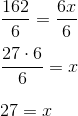## Example Questions

### Example Question #1 : How To Find The Square Of An Integer

The square root of 5184 is:

70

74

73

71

72

72

Explanation:

The easiest way to narrow down a square root from a list is to look at the last number on the squared number – in this case 4 – and compare it to the last number of the answer.

70 * 70 will equal XXX0

71 * 71 will equal XXX1

72 * 72  will equal XXX4

73 * 73 will equal XXX9

74 * 74  will equal XXX(1)6

Therefore 72 is the answer.  Check by multiplying it out.

### Example Question #2 : How To Find The Square Of An Integer

If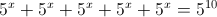, what is the value of x?

9

2

10

1

5

9

Explanation: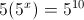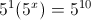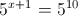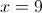### Example Question #3 : How To Find The Square Of An Integer

If x and y are integers and xy + y2 is even, which of the following statements must be true?

I. 3y is odd

II. y/2 is an integer

III. xy is even

II only

I only

I & II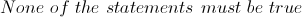I, II, & IIIExplanation:

In order for the original statement to be true, the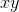andterms must be either both odd or both even. Looking at each of the statements individually,

I. States that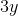is odd, but only odd values multiplied by 3 are odd. Ifwas an even number, the result would be even. Butcan be either odd or even, depending on whatequals. Thus this statement COULD be true but does not HAVE to be true.

II. States that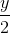is an integer, and since only even numbers are cleanly divided by 2 (odd values result in a fraction) this ensures thatis even. However,can also be odd, so this is a statement that COULD be true but does not HAVE to be true.

III. For exponents, only the base value determines whether it is even or odd - it does not indicate the value of y at all. Only even numbers raised to any power are even, thus, this ensures thatis even. Butcan be odd as well, so this statement COULD be true but does not HAVE to be true.

An example of two integers that will work violate conditions II and III isand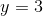.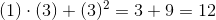, and even number.is not an integer.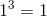is not even.

Furthermore, any combination of 2 even integers will make the original statement true, and violate the Statement I.

### Example Question #4 : How To Find The Square Of An Integer

How many integers from 20 to 80, inclusive, are NOT the square of another integer?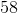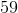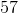Explanation:

First list all the integers between 20 and 80 that are squares of another integer:

52 = 25

62 = 36

72 = 49

82 = 64

In total, there are 61 integers from 20 to 80, inclusive. 61 – 4 = 57

### Example Question #5 : How To Find The Square Of An Integer

Consider the inequality: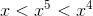Which of the following could be a value of?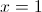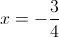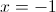There is no possible value for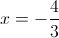Explanation:

Notice how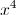is the greatest value. This often means thatis negative as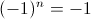when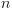is odd and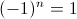whenis even.

Let us examine the first choice,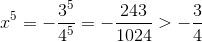This can only be true of a negative value that lies between zero and one.

### Example Question #6 : How To Find The Square Of An Integer

Solve each problem and decide which is the best of the choices given.

Solve for.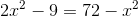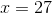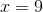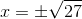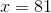Explanation:

To solve foryou have to get all the's on one side and all the numbers on the other side.

Combine like terms, and the result is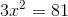.

Divide byon both sides to get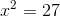.

Finally take the square root of both sides to get.

### Example Question #7 : How To Find The Square Of An Integer

Give the positive solution of the question.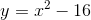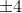Explanation:

This question is testing one's ability to analyze a function algebraically and recognize different but equivalent forms. Identifying properties of functions through analyzing equivalent forms is critical to this concept. Such properties that can be found through analyzing the different forms of a function include finding roots (zeros), extreme values, symmetry, and intercepts.

Knowing the standard and the concept for which it relates to, we can now do the step-by-step process to solve the problem in question.

Step 1: Recognize the general form of the function.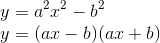This is known as the difference of squares.

Step 2: Identify what is known.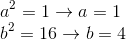Step 3: Substitute the known values into the difference of perfect squares found in step 1.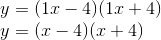To find the positive solution set each binomial equal to zero and solve for x.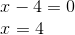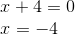Therefore the positive solution is four.

### Example Question #8 : How To Find The Square Of An Integer

If the following are true, solve for.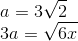Explanation:

Given the two equations, substitute the numerical value ofinto the second equation to solve forSubstituting the numerical value forinto the equation withis as follows.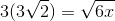From here, distribute the three.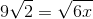Now square both side of the equation.Remember to square both terms within the parentheses. Also, recall that squaring a square root sign cancels them out.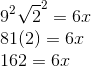To solve fordivide both sides by six.